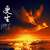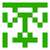1000. A + B ProblemYou are expected to solve this problem till the end of file.
Here is a sample solution for C/C++:

#include <stdio.h>
int main()
{
int a, b;
while (scanf("%d%d", &a, &b) != EOF)
printf("%d\n", a + b);
}

And another sample for Java:

import java.util.Scanner;
public class Main {
public static void main(String args[]) {
Scanner in = new Scanner(System.in);
int a, b;
while (in.hasNextInt()) {
a = in.nextInt();
b = in.nextInt();
System.out.println(a + b);
}
}
}

For other languages, similar way should be done.Hi all! May I ask a question:
Is it possible to enter

1 1
2 3

at a time instead of typing them line by line(I:1 1. O:2. I:2 3. O:5) when testing?
Thank you:)